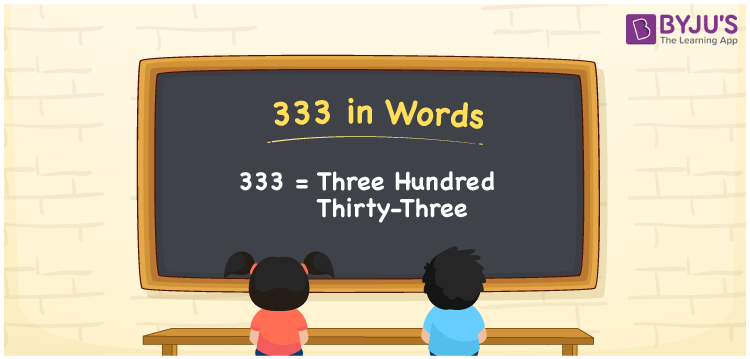# 333 in Words

333 in words can be written as Three Hundred Thirty-three. The concepts like counting numbers which are important from primary education are discussed here in brief. For instance, if you spend Rs. 333 in buying a cap, then you can say that “I bought a cap for Three Hundred Thirty-three Rupees”. The English alphabet is used to write 333 in words. Students can get a clear knowledge of numbers in words with the help of the study materials given at BYJU’S. The number 333 can be read as “Three Hundred Thirty-three” in English.

 333 in words Three Hundred Thirty-three Three Hundred Thirty-three in Numbers 333

## 333 in English Words## How to Write 333 in Words?

Place value chart and expanded form of 333 can be learned easily in this section. There are three digits in the number 333. The place value chart for 333 is provided below for reference to help students understand the concepts effectively.

 Hundreds Tens Ones 3 3 3

The expanded form of 333 is provided below:

3 × Hundred + 3 × Ten + 3 × One

= 3 × 100 + 3 × 10 + 3 × 1

= 333

= Three Hundred Thirty-three

Hence, 333 in words is written as Three Hundred Thirty-three.

333 is a natural number that precedes 334 and succeeds 332.

333 in words – Three Hundred Thirty-three

Is 333 an odd number? – Yes

Is 333 an even number? – No

Is 333 a perfect square number? – No

Is 333 a perfect cube number? – No

Is 333 a prime number? – No

Is 333 a composite number? – Yes

## Frequently Asked Questions on 333 in Words

Q1

### How can 333 be written in words?

333 can be written as “Three Hundred Thirty-three” in words.
Q2

### Is 333 an odd number?

No, 333 is an odd number because it is not divisible by 2.
Q3

### Write Three Hundred Thirty-three in numbers.

Three Hundred Thirty-three can be written in numbers as 333.